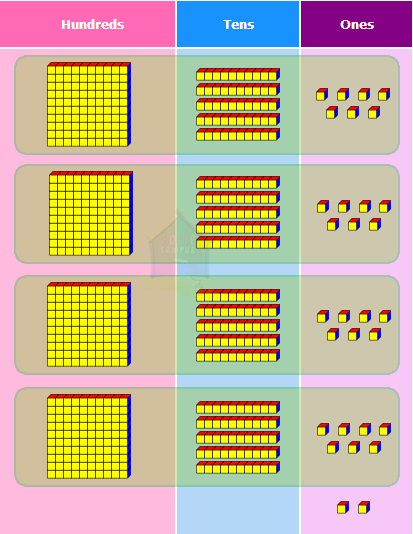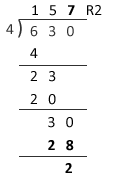## Long Division of Numbers With Remainders

Practice Unlimited Questions

#### 1. Falaq and Zara had 6 roses. They divided the roses equally between them. a) How many roses did each girl receive? b) How many roses were left?a) 6  ÷  2  =  ?
6 ones  ÷  2  =  3 ones with no remainder.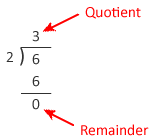b) There were no roses left.

#### 2. 3 friends shared 14 buttons equally among themselves. a) How many buttons did each friend get? b) How many buttons were left?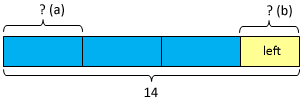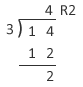a) 14 ones  ÷  3  =  4 ones with a remainder of 2 ones. Each friend got 4 buttons.

b) 2 buttons were left.

#### 3. Mrs. Lee baked 48 muffins. She divided the muffins equally among 4 children. How many muffins did each child receive?Step 1: Divide the tens by 4.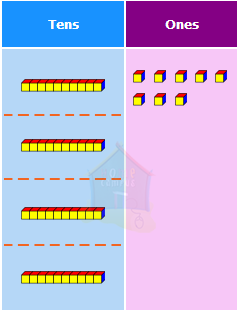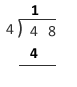4 tens  ÷  4  =  1 ten
Step 2: Divide the ones by 4.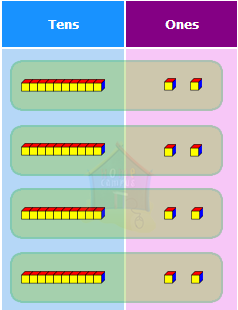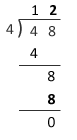8 ones  ÷  4  =  2 ones

#### 4. A fruiterer had 77 apples. He packed them equally into 3 baskets. a) How many apples were there in each basket? b) How many apples were left?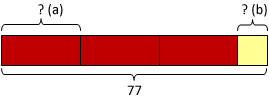Step 1a: Divide the tens by 3.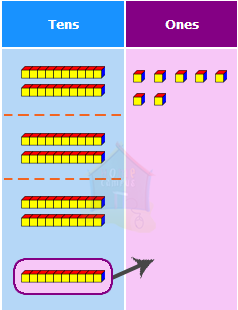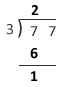7 tens  ÷  3  =  2 tens with a remainder of 1 ten
Step 1b: Regroup the remainder tens.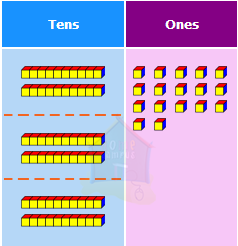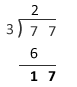1 ten  =  10 ones

10 ones  +  7 ones  =  17 ones
Step 2: Divide the ones by 3.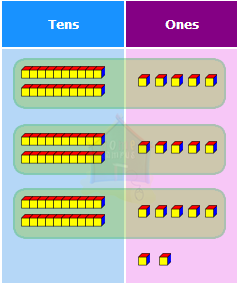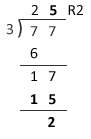17 ones  ÷  3  =  5 ones with a remainder of 2 ones
So, 77  ÷  3  =  25 R 2.

a) There were 25 apples in each basket.

b) 2 apples were left.

#### 5. Jenny collected 630 postcards. She put the postcards equally into 4 albums. a) How many postcards did she put in each album? b) How many postcards were left?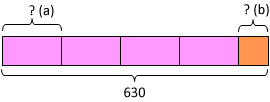Step 1a: Divide the hundreds by 4.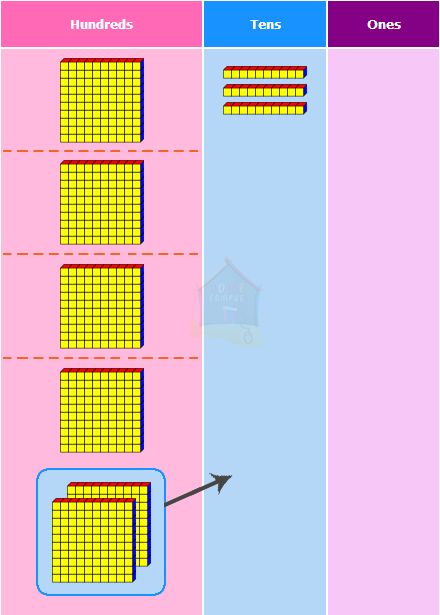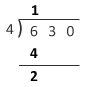6 hundreds  ÷  4  =  1 hundred with a remainder of 2 hundreds
Step 1b: Regroup the remainder hundreds.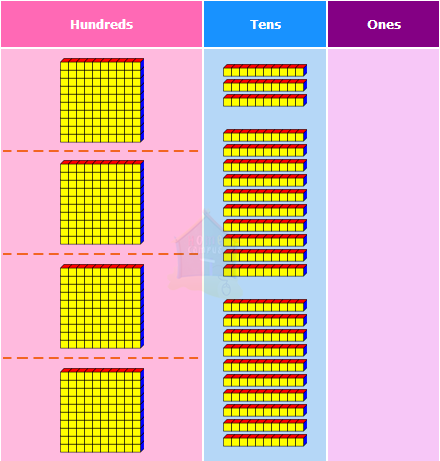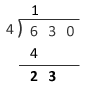2 hundreds  =  20 tens

20 tens  +  3 tens  =  23 tens
Step 2a: Divide the tens by 4.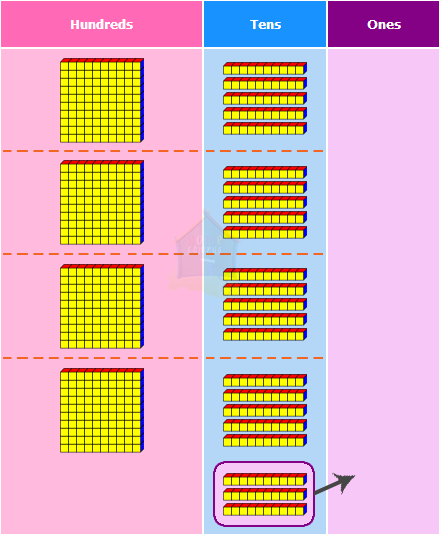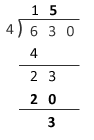23 tens  ÷  4  =  5 tens with a remainder of 3 tens
Step 2b: Regroup the remainder tens.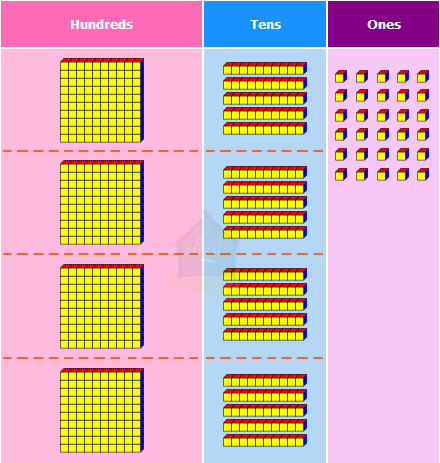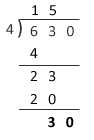3 tens  =  30 ones